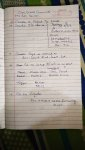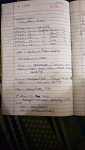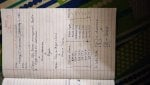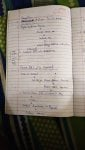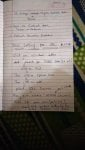# Citect SCADA and SQL Data Transfer

M

#### moh

Hi,

I want to connect to SQL server and get data from it. I show it on textbox in Citect SCADA 2018. I have this code for this task. Data does not show on textbox. Please say your idea for me.
Code:
INT
FUNCTION
GetNames()
INT hSQL;
STRING sName;
INT Status;

hSQL = SQLConnect( "DSN=impaytere_ss" );
IF hSQL <> -1 THEN
Status = SQLExec( hSQL,"SELECT CNAME FROM FETISH WHERE SURNAME = 'Jumpy' " );
IF Status = 0 THEN
WHILE SQLNext( hSQL ) = 0 DO
sName = SQLGetField( hSQL, "CNAME" );

// whatever you wish to do with this data...

END;
SQLEnd( hSQL );

ELSE
Message( "Error", SQLErrMsg(), 48 );
END;

ELSE
Message( "Error", SQLErrMsg(), 48 );
END;
RETURN sName
SQLDisconnect( hSQL );
END

N

#### Nick

A bit late... but where are you calling this function from? Both SQLConnect and SQLExec are BLOCKING functions and cannot be called from foreground tasks. E.g. you cannot call this from a text label's Text expression. This must be called from something like a button press, or an event.

#### shurenu

Code:
FUNCTION SQL()

INT hSQL, nErr;

STRING D1 = Date(10);
STRING D2 = RealToStr(W1,"","");
STRING D3 = RealToStr(W2,"","");
STRING D4 = RealToStr(W3,"","");
STRING D5 = RealToStr(W4,"","");
STRING D6 = RealToStr(W5,"","");

hSQL=SQLConnect("DSN=WWW");

IF hSQL <> -1 THEN

nErr = SQLSet(hSQL, "INSERT INTO Table_2(DATE,DAT1,DAT2,DAT3,DAT4,DAT5)");
nErr = SQLAppend(hSQL, "VALUES('"+D1+"','"+D2+"','"+D3+"','"+D4+"','"+D5+"','"+D6+"')");
nErr = SQLExec(hSQL, "");

IF nErr <> 0 THEN
Message("Information", SQLErrMsg(), 48);
END

nErr = SQLEnd(hSQL);
nErr = SQLDisconnect(hSQL);

ELSE
Message("Information", SQLErrMsg(), 48);
END

END